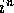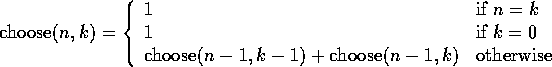# More Recursion

Tom Kelliher, CS18

Feb. 26, 1996

# Raising an Integer to a Power

Computingrecursively

## Method 1Implement this equation

## Method 2Implement this equation

## Comparison

• Is one method ``better'' than the other?
• Define ``better''
• Significance of the difference?

# Homecoming Dilemma

You have n friends, but only enough time to visit k of them

How many different sets of friends can you visit?

Consider 1 friend:

1. Included in some sets: n - 1 remaining friends, with k - 1 to visit
2. Excluded in some sets: n - 1 remaining friends, with k to visit
3. Base cases?
1. m friends, m to visit
2. m friends, 0 to visit

The equation:Write the function

# The Tottering Robot

A certain robot can take steps of length 1 or 2.

Count the number of different ways in which it can take a walk of n steps.

1. How many length 1 walks?
2. Length 2?
3. Length 3?
4. ...
5. Generalize to n in terms of shorter walks

# Practice Problems

1. Write a recursive function to convert a character string of n digits to the corresponding integer number. For example, if the input string is "5274", return the number 5274. Observe that int('5') - int('0') is the number 5. The following demonstrates the idea:
1. 5274 = (527)10 + 4
2. 527 = (52)10 + 7
3. 52 = (5)10 + 2
2. To convert a non-negative decimal integer num to a binary number, repeatedly perform integer division by 2 of successive quotients. The remainders, written in reverse order, are num expressed in binary. Write a recursive function to print a non-negative decimal integer in binary.

Thomas P. Kelliher
Fri Feb 23 11:44:06 EST 1996
Tom Kelliher